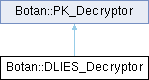Botan 3.0.0-alpha0 Crypto and TLS for C&
Botan::DLIES_Decryptor Class Referencefinal

`#include <dlies.h>`

Inheritance diagram for Botan::DLIES_Decryptor:## Public Member Functions

template<typename Alloc >
secure_vector< uint8_t > decrypt (const std::vector< uint8_t, Alloc > &in) const

secure_vector< uint8_t > decrypt (const uint8_t in[], size_t length) const

secure_vector< uint8_t > decrypt_or_random (const uint8_t in[], size_t length, size_t expected_pt_len, RandomNumberGenerator &rng) const

secure_vector< uint8_t > decrypt_or_random (const uint8_t in[], size_t length, size_t expected_pt_len, RandomNumberGenerator &rng, const uint8_t required_content_bytes[], const uint8_t required_content_offsets[], size_t required_contents) const

DLIES_Decryptor (const DH_PrivateKey &own_priv_key, RandomNumberGenerator &rng, KDF *kdf, Cipher_Mode *cipher, size_t cipher_key_len, MessageAuthenticationCode *mac, size_t mac_key_len=20)

DLIES_Decryptor (const DH_PrivateKey &own_priv_key, RandomNumberGenerator &rng, KDF *kdf, MessageAuthenticationCode *mac, size_t mac_key_len=20)

void set_initialization_vector (const InitializationVector &iv)
Set the initialization vector for the data decryption method. More...

## Detailed Description

DLIES Decryption

Definition at line 98 of file dlies.h.

## ◆ DLIES_Decryptor() [1/2]

 Botan::DLIES_Decryptor::DLIES_Decryptor ( const DH_PrivateKey & own_priv_key, RandomNumberGenerator & rng, KDF * kdf, MessageAuthenticationCode * mac, size_t mac_key_len = `20` )

Stream mode: use KDF to provide a stream of bytes to xor with the message

Parameters
 own_priv_key own (ephemeral) DH private key rng the RNG to use kdf the KDF that should be used mac the MAC function that should be used mac_key_len key length of the MAC function. Default = 20 bytes

input = (ephemeral) public key + ciphertext + tag

Definition at line 130 of file dlies.cpp.

134 :
135 DLIES_Decryptor(own_priv_key, rng, kdf, nullptr, 0, mac, mac_key_length)
136 {}
DLIES_Decryptor(const DH_PrivateKey &own_priv_key, RandomNumberGenerator &rng, KDF *kdf, MessageAuthenticationCode *mac, size_t mac_key_len=20)
Definition: dlies.cpp:130

## ◆ DLIES_Decryptor() [2/2]

 Botan::DLIES_Decryptor::DLIES_Decryptor ( const DH_PrivateKey & own_priv_key, RandomNumberGenerator & rng, KDF * kdf, Cipher_Mode * cipher, size_t cipher_key_len, MessageAuthenticationCode * mac, size_t mac_key_len = `20` )

Block cipher mode

Parameters
 own_priv_key own (ephemeral) DH private key rng the RNG to use kdf the KDF that should be used cipher the block cipher that should be used cipher_key_len the key length of the block cipher mac the MAC function that should be used mac_key_len key length of the MAC function. Default = 20 bytes

input = (ephemeral) public key + ciphertext + tag

Definition at line 110 of file dlies.cpp.

116 :
117 m_pub_key_size(own_priv_key.public_value().size()),
118 m_ka(own_priv_key, rng, "Raw"),
119 m_kdf(kdf),
120 m_cipher(cipher),
121 m_cipher_key_len(cipher_key_len),
122 m_mac(mac),
123 m_mac_keylen(mac_key_length),
124 m_iv()
125 {
128 }
#define BOTAN_ASSERT_NONNULL(ptr)
Definition: assert.h:106

## ◆ decrypt() [1/2]

template<typename Alloc >
 secure_vector< uint8_t > Botan::PK_Decryptor::decrypt ( const std::vector< uint8_t, Alloc > & in ) const
inlineinherited

Same as above, but taking a vector

Parameters
 in the ciphertext
Returns
decrypted message

Definition at line 101 of file pubkey.h.

102 {
103 return decrypt(in.data(), in.size());
104 }
secure_vector< uint8_t > decrypt(const uint8_t in[], size_t length) const
Definition: pubkey.cpp:17

References Botan::CryptoBox::decrypt().

## ◆ decrypt() [2/2]

 secure_vector< uint8_t > Botan::PK_Decryptor::decrypt ( const uint8_t in[], size_t length ) const
inherited

Decrypt a ciphertext, throwing an exception if the input seems to be invalid (eg due to an accidental or malicious error in the ciphertext).

Parameters
 in the ciphertext as a byte array length the length of the above byte array
Returns
decrypted message

Definition at line 17 of file pubkey.cpp.

18 {
20
21 secure_vector<uint8_t> decoded = do_decrypt(valid_mask, in, length);
22
24 throw Decoding_Error("Invalid public key ciphertext, cannot decrypt");
25
26 return decoded;
27 }

Referenced by Botan::KeyPair::encryption_consistency_check().

## ◆ decrypt_or_random() [1/2]

 secure_vector< uint8_t > Botan::PK_Decryptor::decrypt_or_random ( const uint8_t in[], size_t length, size_t expected_pt_len, RandomNumberGenerator & rng ) const
inherited

Decrypt a ciphertext. If the ciphertext is invalid (eg due to invalid padding) or is not the expected length, instead returns a random string of the expected length. Use to avoid oracle attacks, especially against PKCS #1 v1.5 decryption.

Definition at line 78 of file pubkey.cpp.

82 {
83 return decrypt_or_random(in, length, expected_pt_len, rng,
84 nullptr, nullptr, 0);
85 }
secure_vector< uint8_t > decrypt_or_random(const uint8_t in[], size_t length, size_t expected_pt_len, RandomNumberGenerator &rng) const
Definition: pubkey.cpp:78

References Botan::PK_Decryptor::decrypt_or_random().

## ◆ decrypt_or_random() [2/2]

 secure_vector< uint8_t > Botan::PK_Decryptor::decrypt_or_random ( const uint8_t in[], size_t length, size_t expected_pt_len, RandomNumberGenerator & rng, const uint8_t required_content_bytes[], const uint8_t required_content_offsets[], size_t required_contents ) const
inherited

Decrypt a ciphertext. If the ciphertext is invalid (eg due to invalid padding) or is not the expected length, instead returns a random string of the expected length. Use to avoid oracle attacks, especially against PKCS #1 v1.5 decryption.

Additionally checks (also in const time) that: contents[required_content_offsets[i]] == required_content_bytes[i] for 0 <= i < required_contents

Used for example in TLS, which encodes the client version in the content bytes: if there is any timing variation the version check can be used as an oracle to recover the key.

Definition at line 30 of file pubkey.cpp.

37 {
38 const secure_vector<uint8_t> fake_pms = rng.random_vec(expected_pt_len);
39
40 uint8_t decrypt_valid = 0;
41 secure_vector<uint8_t> decoded = do_decrypt(decrypt_valid, in, length);
42
45
46 decoded.resize(expected_pt_len);
47
48 for(size_t i = 0; i != required_contents_length; ++i)
49 {
50 /*
51 These values are chosen by the application and for TLS are constants,
52 so this early failure via assert is fine since we know 0,1 < 48
53
54 If there is a protocol that has content checks on the key where
55 the expected offsets are controllable by the attacker this could
56 still leak.
57
58 Alternately could always reduce the offset modulo the length?
59 */
60
61 const uint8_t exp = required_content_bytes[i];
62 const uint8_t off = required_content_offsets[i];
63
64 BOTAN_ASSERT(off < expected_pt_len, "Offset in range of plaintext");
65
66 auto eq = CT::Mask<uint8_t>::is_equal(decoded[off], exp);
67
69 }
70
73
74 return decoded;
75 }
Definition: assert.h:54
static Mask< T > is_equal(T x, T y)
Definition: ct_utils.h:147
static Mask< T > is_zero(T x)
Definition: ct_utils.h:139

## ◆ set_initialization_vector()

 void Botan::DLIES_Decryptor::set_initialization_vector ( const InitializationVector & iv )
inline

Set the initialization vector for the data decryption method.

Definition at line 140 of file dlies.h.

141 {
142 m_iv = iv;
143 }

The documentation for this class was generated from the following files: